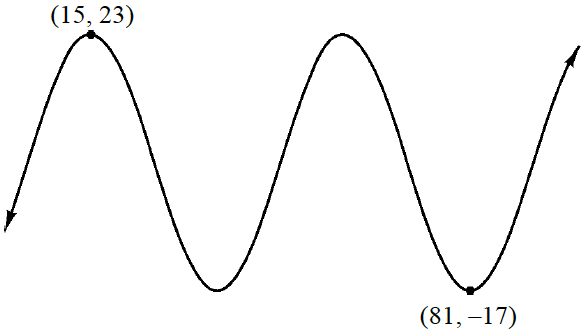### Home > INT3 > Chapter 12 > Lesson 12.2.1 > Problem12-78

12-78.

Each labeled point on the graph below is either a maximum or a minimum.1. What are the period and amplitude of the graph?

Period: How far is it between consecutive maximums or minimums?
Amplitude: How “tall” is the graph? Divide this answer by $2$.

2. Write an equation for the graph.

Find the vertical and horizontal shifts. For the vertical shift, what number is in the middle of $23$ and $−17$?

Review the Math Notes box in Lesson 9.2.4 for help with the general equation of a sine or cosine graph.
In this case, it is more convenient to use cosine because you know a maximum point.

$y=3+20\cos\left( \frac{\pi}{22}(x-15) \right)$

3. Use one of the labeled points to check your equation.

Substitute the values for each of the points into the equation to verify that it is correct.